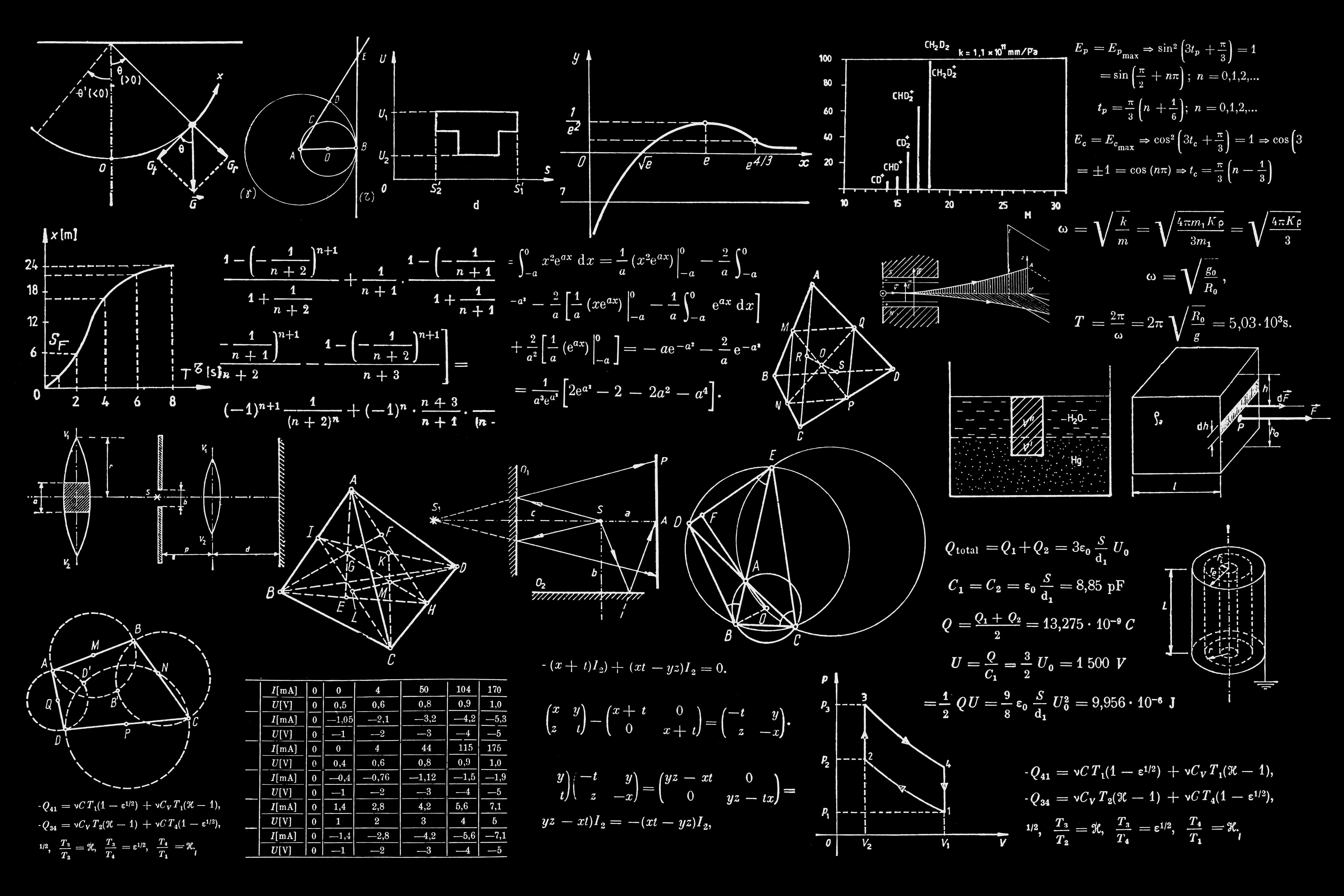# Largest Area in Histogram

##### by Luca Sbardella on August 05, 2020

Given n non-negative integers representing the histogram's bar height where the width of each bar is 1, find the area of largest rectangle in the histogram

This is a visualization of the maximum rectangular area in a histogram. It is the solution of the following problem

Given n non-negative integers representing the histogram's bar height where the width of each bar is 1, find the area of largest rectangle in the histogram.

To solve the problem in O(N) one can use a stack data type:

const largestRectangle = (Height) => {
var stack = [],
max_area = 0,
area,
x1,
x2,
y;

Height.map((h, i) => {
while (stack.length && h < Height[stack[stack.length - 1]]) calc_area(i);
stack.push(i);
});

while (stack.length) calc_area(Height.length);

return [x1, x2, y];

function calc_area(i) {
var j = stack.splice(stack.length - 1);
var t = stack.length ? stack[stack.length - 1] + 1 : 0;
area = Height[j] * (i - t);
if (area > max_area) {
max_area = area;
y = Height[j];
x1 = t;
x2 = i;
}
}
};